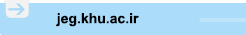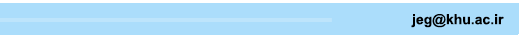[Home ] [Archive]   [ فارسی ]Main About Current Issue Archive Search Submit ContactVolume 13, Issue 1 (Vol. 13, No. 1 2019)2019, 13(1): 155-184 Back to browse issues page
Proposed Theoretical Equations for Estimating the Compressive and Tensile Strength of Unreinforced and Reinforced Soil and Soil-Cement
1- , raeesi@ut.ac.ir
Abstract:   (3153 Views)
Introduction
Improving the mechanical behavior of clay soil by stabilization agents is a mean of fulfilling geotechnical design criteria. The method of stabilization can be divided into chemical, mechanical, or a combination of both methods. Chemical stabilization is performed by adding chemical agents such as cement, lime or fly ash to the soil (Bahar et al., 2004). Soil reinforcement is one of the mechanical methods that is used for improving the behavior of soils (Tang et al., 2007). Reinforcement of soil achieved by either inclusion of strips, bars, grids and etc. within a soil mass in a preferred direction or mixing discrete fibers randomly with a soil mass.
Mixing of cement with soil is made a production that is called soil-cement and results in chemical reaction between soil, cement, and water. The compressive strength of soil-cement is increased by increasing the cement content and this leads to brittle behavior or sudden failure. On the other hand, by increasing the cement to soil ratio for cohesive soils, shrinkage micro-cracks may develop in the soil as a result of the loss of water content during drying or hydration of cement. Therefore, if the tensile strength of these materials is not sufficient cracks will develop under loading and damage will be resulted (Khattak and Alrashidi, 2006). Consoli et al. (2003) and Tang et al. (2007) indicated that adding the fiber to soil can prevent from occurrence of these cracks and increases the tensile strength of the soil.
The focus of this paper is on the statistical analysis of the results and development of regression models. Regression relationships are developed based on the experimental results that were presented by Estabragh et al. (2017). These relationships relate the compressive and tensile strengths of the soil to percent of used fiber, cement and curing time.
Material and methods of testing
Unconfined compression and tensile strength tests were carried on unreinforced and reinforced soil, soil cement according to ASTM standards. Samples of soil-cement were made by mixing a clay soil and two different weight percent of cement (8 and 10%). Reinforced soil samples were also prepared by mixing 0.5 and 1 weight percent of Polypropylene fibers with 10, 15, 20 and 25 mm lengths. The dry unit weight and water content of prepared samples were the same as optimum water content and maximum dry unit weight that were resulted from standard compaction test. The compressive and tensile strength tests were conducted on the samples by considering the curing time according to ASTM standards until the failure of the sample is achieved.
Results and discussion
The experimental tests showed that reinforcement of the soil and soil cement increase the peak compressive and tensile strength. The peak compressive strength of reinforced soil is increased by increasing the fiber content at a constant length of the fiber. It can be said that by increasing the percent of fiber, the number of fibers in the sample is increased and contact between soil particle and fibers is increased which result in increase in the strength (Maher 1994). However, by increasing the length of the constant fiber inclusion there will be no significant increase in strength because the number of shorter fiber is more than longer fiber in a specific sample (Ahmad et al., 2010). Inclusion of fibers can greatly increase the tensile strength of clay soil. In addition to reinforcement of soil cement showed the same trend. When fiber is added to soil cement, the surface of fiber adheres to the hydration products of cement and some clay particle. Therefore, this combination increases the efficiency of load transfer from the composition to the fibers which increase the peak strength (Tang et al., 2007). In addition, the tensile strength shows the same trend.
Based on the experimental data on the behavior of a randomly reinforced clay soil and soil cement multiple regression models (linear and non-linear) were developed for calculating the peak compressive and tensile strength (dependent variables) based on the value of the coefficient of determination (R2). The proposed regression models were functions of independent variables including weight percent of fiber, length of fiber (length/diameter of fiber), weight percent of cement, and curing time. Finally, the comparison is made between the predicted results from proposed models and experimental results. In order to investigate the model accuracy, the Root Mean Square Error (RMSE) and Normalized Root Mean Square Error (NRMSE) are used.
The Multiple Linear Regression models (MLR) was very suitable for the study of the effect of independent variables on the quantitative analytic dependent variable. The NRSME for peak compressive and tensile strength is was 3.59% and 5.11% respectively for these models. Also, the Multiple Nonlinear Regression models (MNLR) had a much lower error than the linear model because of the quadratic equation, the equation will be able to predict the increase and decrease of the output variable in terms of the increase of the independent input variable. Therefore, The NRMSE for peak compressive and tensile strength was 1.02% and 4.04% for MNLR models respectively.
Conclusion
The following conclusions can be drawn from this study:
- The strength of reinforced soil and soil cement is increased by increasing the fiber content.
- Increasing the length of the fibers in the soil and soil cement has no significant effect on increasing the peak compressive strength, but it will be effective in increasing the tensile strength.
- The Multiple Nonlinear Regression models (MNLR) have more accuracy for prediction of output variable (peak strength) because of lower normalized root mean square error../files/site1/files/131/7Extended_Abstract.pdf

Keywords: Artificial fiber, randomly reinforced, soil cement, soil strength, regression model
Type of Study: s | Subject: Geotecnic
Received: 2017/07/16 | Accepted: 2018/04/30 | Published: 2018/08/20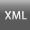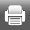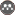Rights and permissionsThis work is licensed under a Creative Commons Attribution-NonCommercial 4.0 International License.